# Breadth First Search or BFS for a Graph

Breadth First Traversal (or Search) for a graph is similar to Breadth First Traversal of a tree (See method 2 of this post). The only catch here is, unlike trees, graphs may contain cycles, so we may come to the same node again. To avoid processing a node more than once, we use a boolean visited array. For simplicity, it is assumed that all vertices are reachable from the starting vertex.
For example, in the following graph, we start traversal from vertex 2. When we come to vertex 0, we look for all adjacent vertices of it. 2 is also an adjacent vertex of 0. If we don’t mark visited vertices, then 2 will be processed again and it will become a non-terminating process. A Breadth First Traversal of the following graph is 2, 0, 3, 1.## Recommended: Please solve it on “PRACTICE ” first, before moving on to the solution.

Following are the implementations of simple Breadth First Traversal from a given source.

The implementation uses adjacency list representation of graphs. STL‘s list container is used to store lists of adjacent nodes and queue of nodes needed for BFS traversal.

## C++

 `// Program to print BFS traversal from a given ` `// source vertex. BFS(int s) traverses vertices  ` `// reachable from s. ` `#include ` `#include ` ` `  `using` `namespace` `std; ` ` `  `// This class represents a directed graph using ` `// adjacency list representation ` `class` `Graph ` `{ ` `    ``int` `V;    ``// No. of vertices ` ` `  `    ``// Pointer to an array containing adjacency ` `    ``// lists ` `    ``list<``int``> *adj;    ` `public``: ` `    ``Graph(``int` `V);  ``// Constructor ` ` `  `    ``// function to add an edge to graph ` `    ``void` `addEdge(``int` `v, ``int` `w);  ` ` `  `    ``// prints BFS traversal from a given source s ` `    ``void` `BFS(``int` `s);   ` `}; ` ` `  `Graph::Graph(``int` `V) ` `{ ` `    ``this``->V = V; ` `    ``adj = ``new` `list<``int``>[V]; ` `} ` ` `  `void` `Graph::addEdge(``int` `v, ``int` `w) ` `{ ` `    ``adj[v].push_back(w); ``// Add w to v’s list. ` `} ` ` `  `void` `Graph::BFS(``int` `s) ` `{ ` `    ``// Mark all the vertices as not visited ` `    ``bool` `*visited = ``new` `bool``[V]; ` `    ``for``(``int` `i = 0; i < V; i++) ` `        ``visited[i] = ``false``; ` ` `  `    ``// Create a queue for BFS ` `    ``list<``int``> queue; ` ` `  `    ``// Mark the current node as visited and enqueue it ` `    ``visited[s] = ``true``; ` `    ``queue.push_back(s); ` ` `  `    ``// 'i' will be used to get all adjacent ` `    ``// vertices of a vertex ` `    ``list<``int``>::iterator i; ` ` `  `    ``while``(!queue.empty()) ` `    ``{ ` `        ``// Dequeue a vertex from queue and print it ` `        ``s = queue.front(); ` `        ``cout << s << ``" "``; ` `        ``queue.pop_front(); ` ` `  `        ``// Get all adjacent vertices of the dequeued ` `        ``// vertex s. If a adjacent has not been visited,  ` `        ``// then mark it visited and enqueue it ` `        ``for` `(i = adj[s].begin(); i != adj[s].end(); ++i) ` `        ``{ ` `            ``if` `(!visited[*i]) ` `            ``{ ` `                ``visited[*i] = ``true``; ` `                ``queue.push_back(*i); ` `            ``} ` `        ``} ` `    ``} ` `} ` ` `  `// Driver program to test methods of graph class ` `int` `main() ` `{ ` `    ``// Create a graph given in the above diagram ` `    ``Graph g(4); ` `    ``g.addEdge(0, 1); ` `    ``g.addEdge(0, 2); ` `    ``g.addEdge(1, 2); ` `    ``g.addEdge(2, 0); ` `    ``g.addEdge(2, 3); ` `    ``g.addEdge(3, 3); ` ` `  `    ``cout << ``"Following is Breadth First Traversal "` `         ``<< ``"(starting from vertex 2) \n"``; ` `    ``g.BFS(2); ` ` `  `    ``return` `0; ` `} `

## Java

 `// Java program to print BFS traversal from a given source vertex. ` `// BFS(int s) traverses vertices reachable from s. ` `import` `java.io.*; ` `import` `java.util.*; ` ` `  `// This class represents a directed graph using adjacency list ` `// representation ` `class` `Graph ` `{ ` `    ``private` `int` `V;   ``// No. of vertices ` `    ``private` `LinkedList adj[]; ``//Adjacency Lists ` ` `  `    ``// Constructor ` `    ``Graph(``int` `v) ` `    ``{ ` `        ``V = v; ` `        ``adj = ``new` `LinkedList[v]; ` `        ``for` `(``int` `i=``0``; i queue = ``new` `LinkedList(); ` ` `  `        ``// Mark the current node as visited and enqueue it ` `        ``visited[s]=``true``; ` `        ``queue.add(s); ` ` `  `        ``while` `(queue.size() != ``0``) ` `        ``{ ` `            ``// Dequeue a vertex from queue and print it ` `            ``s = queue.poll(); ` `            ``System.out.print(s+``" "``); ` ` `  `            ``// Get all adjacent vertices of the dequeued vertex s ` `            ``// If a adjacent has not been visited, then mark it ` `            ``// visited and enqueue it ` `            ``Iterator i = adj[s].listIterator(); ` `            ``while` `(i.hasNext()) ` `            ``{ ` `                ``int` `n = i.next(); ` `                ``if` `(!visited[n]) ` `                ``{ ` `                    ``visited[n] = ``true``; ` `                    ``queue.add(n); ` `                ``} ` `            ``} ` `        ``} ` `    ``} ` ` `  `    ``// Driver method to ` `    ``public` `static` `void` `main(String args[]) ` `    ``{ ` `        ``Graph g = ``new` `Graph(``4``); ` ` `  `        ``g.addEdge(``0``, ``1``); ` `        ``g.addEdge(``0``, ``2``); ` `        ``g.addEdge(``1``, ``2``); ` `        ``g.addEdge(``2``, ``0``); ` `        ``g.addEdge(``2``, ``3``); ` `        ``g.addEdge(``3``, ``3``); ` ` `  `        ``System.out.println(``"Following is Breadth First Traversal "``+ ` `                           ``"(starting from vertex 2)"``); ` ` `  `        ``g.BFS(``2``); ` `    ``} ` `} ` `// This code is contributed by Aakash Hasija `

## Python3

 `# Python3 Program to print BFS traversal ` `# from a given source vertex. BFS(int s) ` `# traverses vertices reachable from s. ` `from` `collections ``import` `defaultdict ` ` `  `# This class represents a directed graph ` `# using adjacency list representation ` `class` `Graph: ` ` `  `    ``# Constructor ` `    ``def` `__init__(``self``): ` ` `  `        ``# default dictionary to store graph ` `        ``self``.graph ``=` `defaultdict(``list``) ` ` `  `    ``# function to add an edge to graph ` `    ``def` `addEdge(``self``,u,v): ` `        ``self``.graph[u].append(v) ` ` `  `    ``# Function to print a BFS of graph ` `    ``def` `BFS(``self``, s): ` ` `  `        ``# Mark all the vertices as not visited ` `        ``visited ``=` `[``False``] ``*` `(``len``(``self``.graph)) ` ` `  `        ``# Create a queue for BFS ` `        ``queue ``=` `[] ` ` `  `        ``# Mark the source node as  ` `        ``# visited and enqueue it ` `        ``queue.append(s) ` `        ``visited[s] ``=` `True` ` `  `        ``while` `queue: ` ` `  `            ``# Dequeue a vertex from  ` `            ``# queue and print it ` `            ``s ``=` `queue.pop(``0``) ` `            ``print` `(s, end ``=` `" "``) ` ` `  `            ``# Get all adjacent vertices of the ` `            ``# dequeued vertex s. If a adjacent ` `            ``# has not been visited, then mark it ` `            ``# visited and enqueue it ` `            ``for` `i ``in` `self``.graph[s]: ` `                ``if` `visited[i] ``=``=` `False``: ` `                    ``queue.append(i) ` `                    ``visited[i] ``=` `True` ` `  `# Driver code ` ` `  `# Create a graph given in ` `# the above diagram ` `g ``=` `Graph() ` `g.addEdge(``0``, ``1``) ` `g.addEdge(``0``, ``2``) ` `g.addEdge(``1``, ``2``) ` `g.addEdge(``2``, ``0``) ` `g.addEdge(``2``, ``3``) ` `g.addEdge(``3``, ``3``) ` ` `  `print` `(``"Following is Breadth First Traversal"` `                  ``" (starting from vertex 2)"``) ` `g.BFS(``2``) ` ` `  `# This code is contributed by Neelam Yadav `

## C#

 `// C# program to print BFS traversal  ` `// from a given source vertex.  ` `// BFS(int s) traverses vertices  ` `// reachable from s.  ` `using` `System; ` `using` `System.Collections.Generic; ` `using` `System.Linq; ` `using` `System.Text; ` ` `  `// This class represents a directed  ` `// graph using adjacency list  ` `// representation  ` `class` `Graph{ ` `     `  `// No. of vertices      ` `private` `int` `_V;  ` ` `  `//Adjacency Lists  ` `LinkedList<``int``>[] _adj;  ` ` `  `public` `Graph(``int` `V) ` `{ ` `    ``_adj = ``new` `LinkedList<``int``>[V]; ` `    ``for``(``int` `i = 0; i < _adj.Length; i++) ` `    ``{ ` `        ``_adj[i] = ``new` `LinkedList<``int``>(); ` `    ``} ` `    ``_V = V; ` `} ` ` `  `// Function to add an edge into the graph  ` `public` `void` `AddEdge(``int` `v, ``int` `w) ` `{          ` `    ``_adj[v].AddLast(w); ` ` `  `} ` ` `  `// Prints BFS traversal from a given source s  ` `public` `void` `BFS(``int` `s) ` `{ ` `     `  `    ``// Mark all the vertices as not ` `    ``// visited(By default set as false)  ` `    ``bool``[] visited = ``new` `bool``[_V]; ` `    ``for``(``int` `i = 0; i < _V; i++) ` `        ``visited[i] = ``false``; ` `     `  `    ``// Create a queue for BFS  ` `    ``LinkedList<``int``> queue = ``new` `LinkedList<``int``>(); ` `     `  `    ``// Mark the current node as  ` `    ``// visited and enqueue it  ` `    ``visited[s] = ``true``; ` `    ``queue.AddLast(s);          ` ` `  `    ``while``(queue.Any()) ` `    ``{ ` `         `  `        ``// Dequeue a vertex from queue  ` `        ``// and print it ` `        ``s = queue.First(); ` `        ``Console.Write(s + ``" "` `); ` `        ``queue.RemoveFirst(); ` `         `  `        ``// Get all adjacent vertices of the  ` `        ``// dequeued vertex s. If a adjacent ` `        ``// has not been visited, then mark it  ` `        ``// visited and enqueue it  ` `        ``LinkedList<``int``> list = _adj[s]; ` ` `  `        ``foreach` `(``var` `val ``in` `list)              ` `        ``{ ` `            ``if` `(!visited[val]) ` `            ``{ ` `                ``visited[val] = ``true``; ` `                ``queue.AddLast(val); ` `            ``} ` `        ``} ` `    ``} ` `} ` ` `  `// Driver code ` `static` `void` `Main(``string``[] args) ` `{ ` `    ``Graph g = ``new` `Graph(4);  ` `     `  `    ``g.AddEdge(0, 1);  ` `    ``g.AddEdge(0, 2);  ` `    ``g.AddEdge(1, 2);  ` `    ``g.AddEdge(2, 0);  ` `    ``g.AddEdge(2, 3);  ` `    ``g.AddEdge(3, 3); ` `     `  `    ``Console.Write(``"Following is Breadth First "` `+ ` `                  ``"Traversal(starting from "` `+ ` `                  ``"vertex 2)\n"``); ` `    ``g.BFS(2); ` `} ` `} ` ` `  `// This code is contibuted by anv89 `

Output:

```Following is Breadth First Traversal (starting from vertex 2)
2 0 3 1
```

Illustration :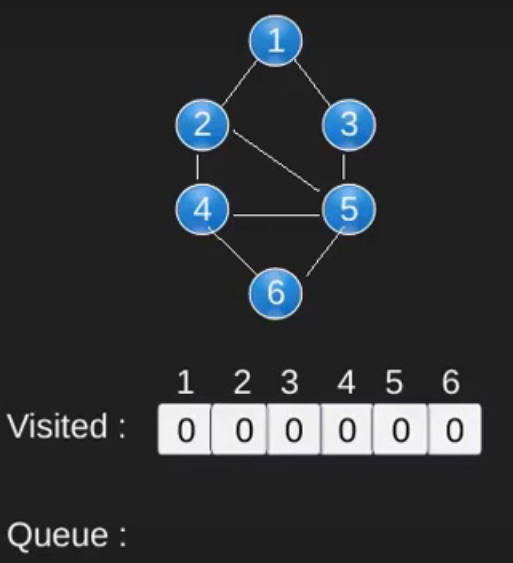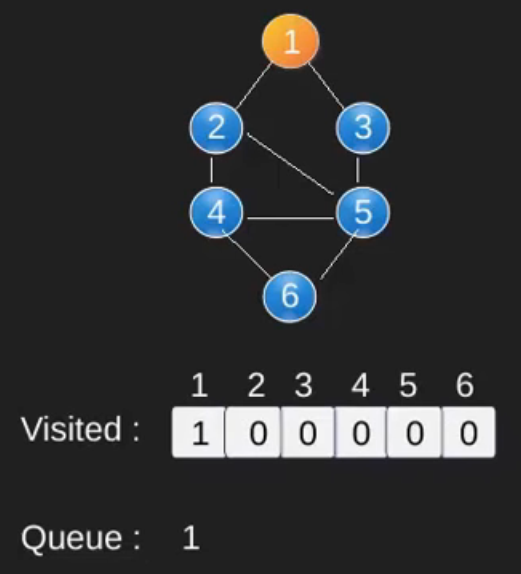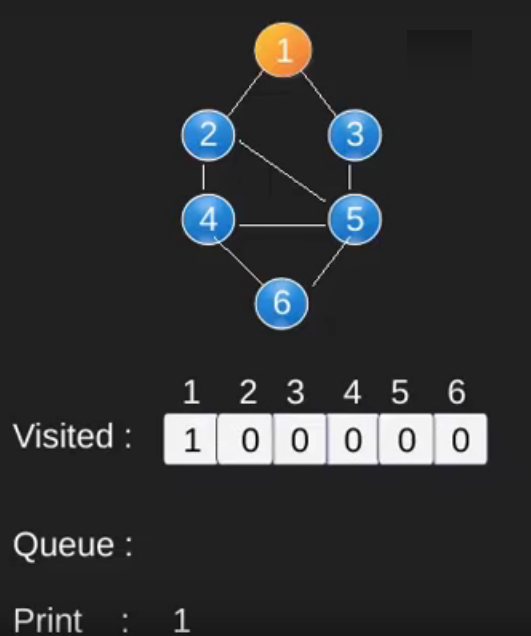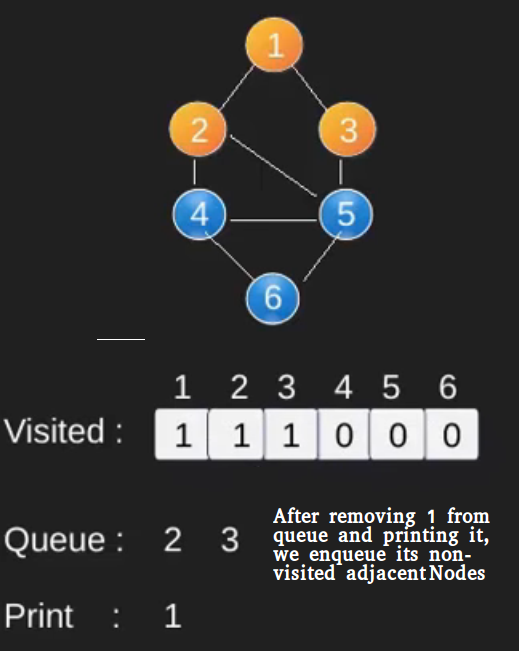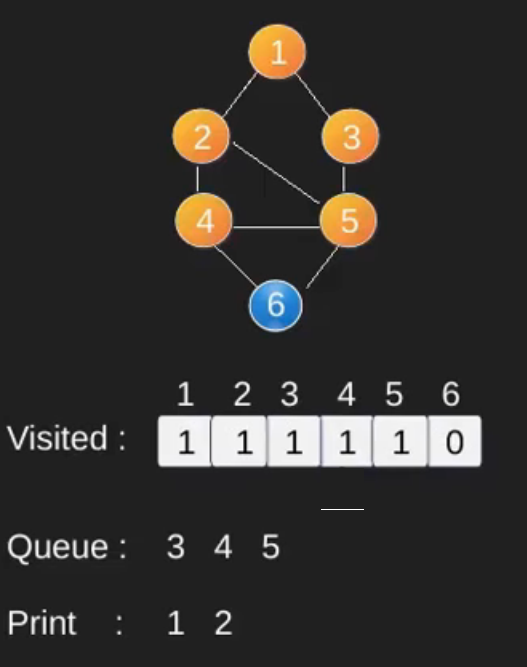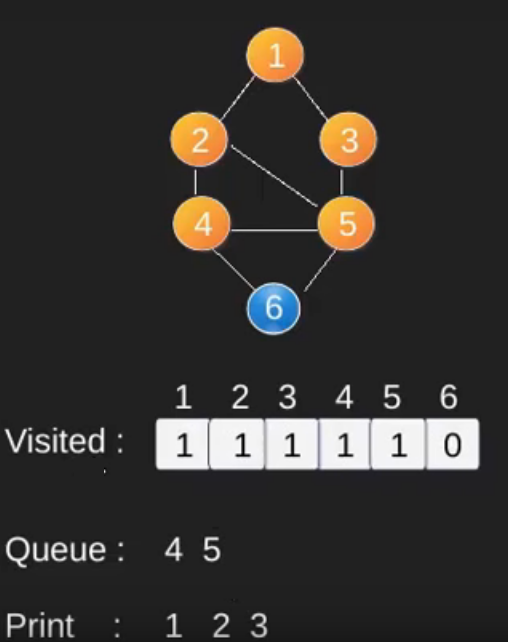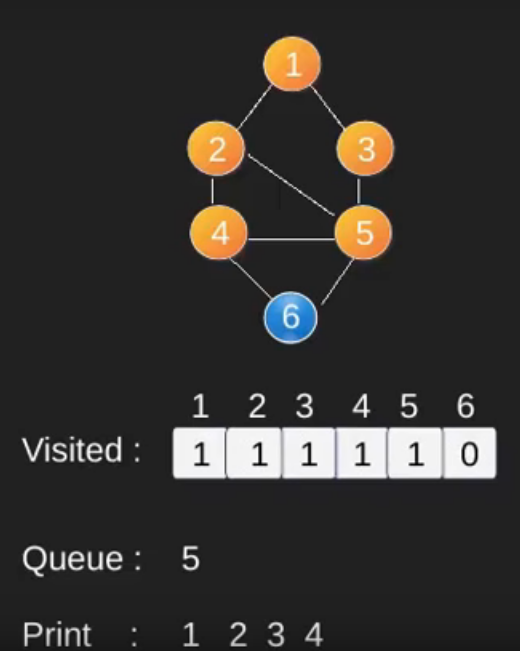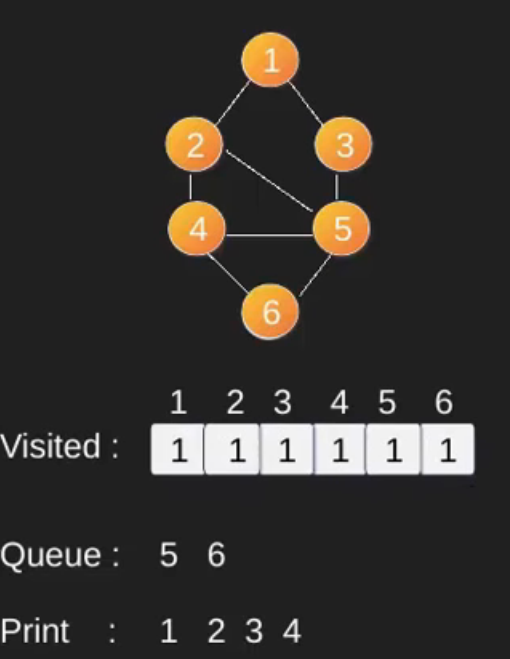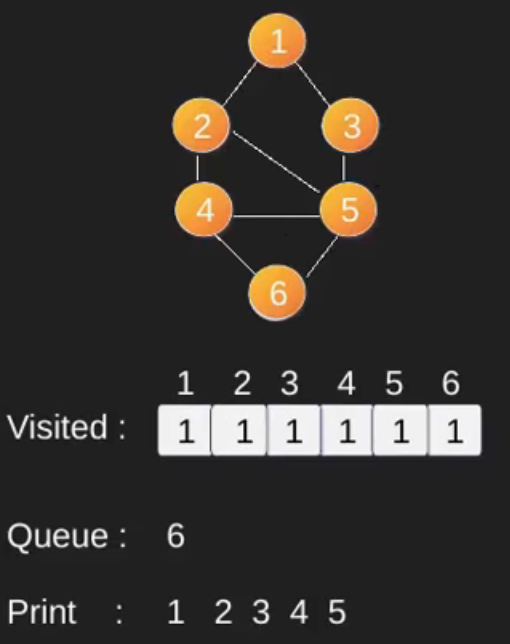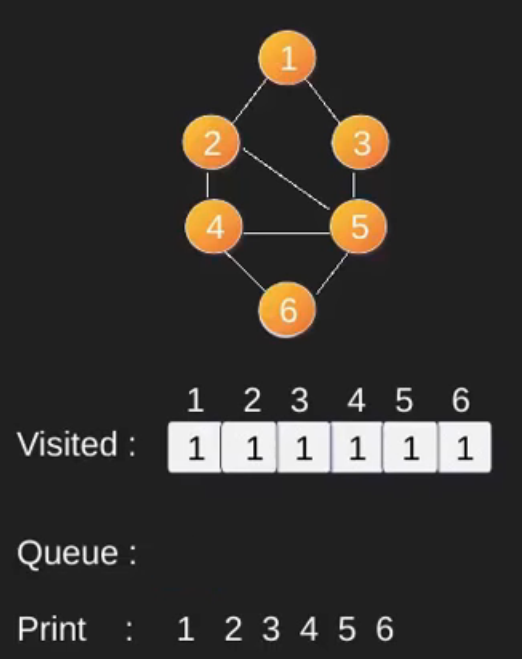Note that the above code traverses only the vertices reachable from a given source vertex. All the vertices may not be reachable from a given vertex (example Disconnected graph). To print all the vertices, we can modify the BFS function to do traversal starting from all nodes one by one (Like the DFS modified version) .

Time Complexity: O(V+E) where V is number of vertices in the graph and E is number of edges in the graph.

You may like to see below also :

Attention reader! Don’t stop learning now. Get hold of all the important DSA concepts with the DSA Self Paced Course at a student-friendly price and become industry ready.

My Personal Notes arrow_drop_up

Improved By : anvudemy1

Article Tags :
Practice Tags :

141

Please write to us at contribute@geeksforgeeks.org to report any issue with the above content.